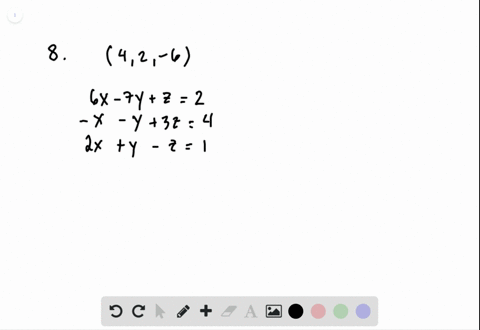Enroll in one of our FREE online STEM summer camps. Space is limited so join now!View Summer Courses### For the following exercises, determine whether th…

01:10Southern Methodist University

Need more help? Fill out this quick form to get professional live tutoring.

Get live tutoring
Problem 7

For the following exercises, determine whether the ordered triple given is the solution to the system of equations.
\begin{aligned} 6 x-y+3 z &=6 \\ 3 x+5 y+2 z &=0 \quad \text { and }(3,-3,-5) \\ x+y &=0 \end{aligned}

the ordered triple $(3,-3,-5)$ is not a solution to the system.

## Discussion

You must be signed in to discuss.

## Video Transcript

okay for this problem, we need to determine if this ordered Triple three negative three. Negative five x y z is a solution to this system of equations. Okay? And to determine this, we need to plug in X, y and Z into each of the equations To be sure that it satisfies that equation. We're gonna start with the 1st 1 and plug in three for X three. Negative three for why? And negative five for sea and see if this equal six. So we have 18 plus three minus 15 which does, in fact, equal six. This is not enough to assume that it satisfies the entire system of equations, so we need to check it in the next equation. And so in the second line, I'm gonna plug in again. Three for X negative three for why? And negative five for sea. And see if this equal zero nine minus 15 minus 10 will not equal zero. And so it does not satisfy the second equation. At this point, we do not need to check the third equation as soon as it fails one of the equations than we know that it is not a solution to the system. Thank you for watching